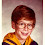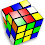## Friday, February 15, 2008

### This Number is a Two-Timer...

Here's a math puzzle for you to ponder. Feel free to post your answer in the comments.
Q: A positive integer has a unknown number of digits but it ends in a two. If the two is moved to the front of the number the new number will be exactly double the old. What is the number?

1.I played around with it but I keep ending up with equations that look something like this:

2000A+200B+20C+2=2000+100A+10B+C
1900A + 190B + 19C = 1998
19(100A + 10B + C) = 1998

1998 not being evenly divisible by 19, there doesn't seem to be any integer combination, even if you use a zero, that will get the job done. The same holds true for four variables, etc.

Guess I'm on the wrong track?

2.Ben,

It has an unknown number of digits which is why I included an ellipsis ...

3.You tried 3 or 4 variables but have you gone higher?

4.Well, the first digit is a 1. The next-to-last digit is a 4. That fulfills the basic requirements given by the problem statement.

5.You're on the right track when you start with "the second to last digit must be 4". You know that because you know the last digit is 2, and doubling a number that ends in 2 gives a number that ends in 4.

Now continue from there. If you know it ends in 42, then the next digit on the left of 4 must be 8. This is because doubling any number that ends in 42 gives a number that ends in 84. Hence the last 3 digits are 842

Keep going with this reasoning. The trick is not to give up!

6.I did it in 18 places. Any takers??

7.105263157894736842

8.Don and Pantheist are exactly correct. The last digit is 2 which will get moved to the front. Therefore the next digit has to be double this:
...42
Then double the 4:
...842
Then double the 8 (remember the carry):
...(1)6842
Then double the 6 and add the carry (13), that gets you another carry:
...(1)36842
Double the 3 and add the carry (7)
...736842
Double the 7 (remember the carry)
...(1)4736842
Double the 4 and add the carry (9)
...94736842
Double the 9 and remember the carry
...(1)894736842
Double the 8 and add the carry (17), remember the new carry:
...(1)7894736842
etc.
...(1)57894736842
...(1)157894736842
...3157894736842
...63157894736842
..(1)263157894736842
.5263157894736842
(1)05263157894736842
Now when you double you get back to the digit 2 so you are done.

105,263,157,894,736,842

P.S. This is the shortest number. You could actually repeat this sequence of digits as much as you like.

For NPR puzzle posts, don't post the answer or any hints that could lead to the answer before the deadline (usually Thursday at 3pm ET). If you know the answer, submit it to NPR, but don't give it away here.

You may provide indirect hints to the answer to show you know it, but make sure they don't assist with solving. You can openly discuss your hints and the answer after the deadline. Thank you.